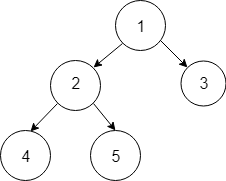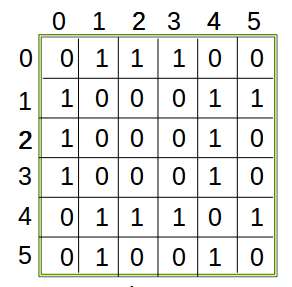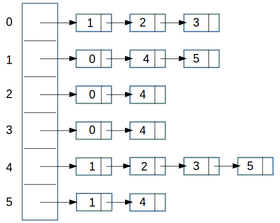×

Search anything:

# Introduction to Tree Data Structure

#### Data Structures tree data structure AlgorithmsGet this book -> Problems on Array: For Interviews and Competitive Programming

In this article, we have presented a detailed introduction to Tree Data Structure. This will quickly give you the idea of Tree, how it is implemented and the different types that are used.

1. What is Tree Data Structure?
2. Generalization of Tree
3. Implementation of Tree Data Structures
4. Types of Trees

Pre-requisites:

## What is Tree Data Structure?

Tree Data Structure is a Data Structure where:

• One element is connected to M or less than M different elements
• There is one element E1 to which no other element points to. This is the root of the Tree (starting point).
• There are multiple elements which do not point to any other elements. These are known as leaf nodes.
• All nodes are reachable from the root node that is if you start from the root node, you can reach every node if you follow a specific path.
• There are no loops: that is in a sequence of nodes (a1, a2, a3, ..., aj) no two nodes are same.

This is how you can visualize a Tree:A Tree is a collection of data where data are interconnected in one direction (think of time which flows in forward direction but there can be branches).

A Tree Data Structure can be visualized as a Tree (in real life). The trunk starts from a root node and the branches of the tree are different connections. Nodes exist at the point where a branch split. The leaves are leaf nodes of the Tree as these are end points.

There are several varieties of a Tree Data Structure.

## Generalization of Tree

A Tree Data Structure is a special case a Graph Data Structure. A Graph Data Structure can have loops while a Tree is not permitted to have a loop.

Hence, a Tree is a more restricted version of a Graph.

An Array Data Structure can be visualized as a special case of tree as well where:

• Each element points to only one other element.
• There is only one leaf node.

Hence, a Tree is a generalization of Array.

To summarize the idea:

``````Array -> Tree -> Graph
``````

## Implementation of Tree Data Structures

There are multiple ways to implement a Tree Data Structure. The best technique depends on the problem we are solving. Some implementation approaches include:

• Custom Class definition for Tree
• Data Structures for Graph

### Custom Class definition for Tree

In OOP approach, a class is defined where an unit is a node and each node point to other nodes.

Following is a sample class definition of Binary Tree (a specific type of Tree):

``````public class BinaryTree {
public static class Node {
int data;
Node left;
Node right;
public Node(int data) {
this.data = data;
this.left = null;
this.right = null;
}
}
public Node root;
}
``````

Array / Linked List can be used for implementing Tree data structure as well. For example, Heap which is a specific type of Tree is implemented using Array by default.

One approach is:

• Element at index I has two children which are at index 2I+1 and 2I+2.
• Root of the Tree is at index 0.

### Data Structures for Graph

As Graph is a generalization for Tree, Data Structures for Graph can be easily used for Tree Data Structures. Two approaches are:

A Adjacency Matrix is a NxN binary matrix in which value of [i,j]th cell is 1 if there exists an edge originating from ith vertex and terminating to jth vertex, otherwise the value is 0.

This is how an adjacency matrix looks like:Adjacency List is an array of seperate lists. Each element of array is a list of corresponding neighbour(or directly connected) vertices.In other words ith list of Adjacency List is a list of all those vertices which is directly connected to ith vertex.

This is how an Adjacency List looks like:## Types of Trees

There are different types of Tree Data Structures and each is used for different problems. Some of the types are:

• Binary Tree
• Binary Search Tree
• Min/ Max Heap
• Self Balancing Binary Tree
• B-Tree

#### Binary Tree

Binary Tree is a Tree where each node has at most 2 children nodes. This is the most common tree that is used and visualized when one talks about tree.

#### Binary Search Tree (BST)

Binary Search Tree (BST) is a specialized version of Binary Tree where:

• the element on the root is greater than the element on the left child node
• the element on the root is less than the element on the right child node

Binary Search Tree has a specific ordering of elements and hence, are frequently used in problems that benefit from partial ordering.

#### Min/ Max Heap

Min/ Max Heap is a specialized version of Binary Tree and is similar to Binary Search Tree. The difference is:

• Element on the root node is greater than the element on the left and right child nodes for Max Heap.
• Similarly, for Min Heap, Element on the root node is less than the element on the left and right child nodes

Heap is often, implemented using Array in contrast to a custom data structure. This also has partial ordering and is the basis of a sorting technique known as Heap Sort.

#### Self Balancing Tree

Self Balancing Tree is a Tree whose height is of the order of logN where N is the number of nodes in the tree. Height is the maximum length from root to leaf node. Some types of Self Balancing Tree are:

• 2-3 tree
• Red-Black Tree
• AVL tree
• AA tree

If a Tree is Balanced, it opens up a lot of opportunities and problems are solved efficiently. A Tree which is unbalanced to the extreme is same as an Array performance and implementation wise. Moveover, Self Balancing Tree demonstrate that keeping Tree balanced does not incur addition cost on average.

#### B-Tree

B-Tree is a generalization of Binary Tree where each node has any number of children nodes. This is used in indexing applications.

This is just the introduction. There are several other types of Trees that are widely used. With this article at OpenGenus, you must have a strong hold on Tree Data Structures.#### Ue Kiao, PhD

Ue Kiao is a Technical Author and Software Developer with B. Sc in Computer Science at National Taiwan University and PhD in Algorithms at Tokyo Institute of Technology | Researcher at TaoBao

Introduction to Tree Data Structure